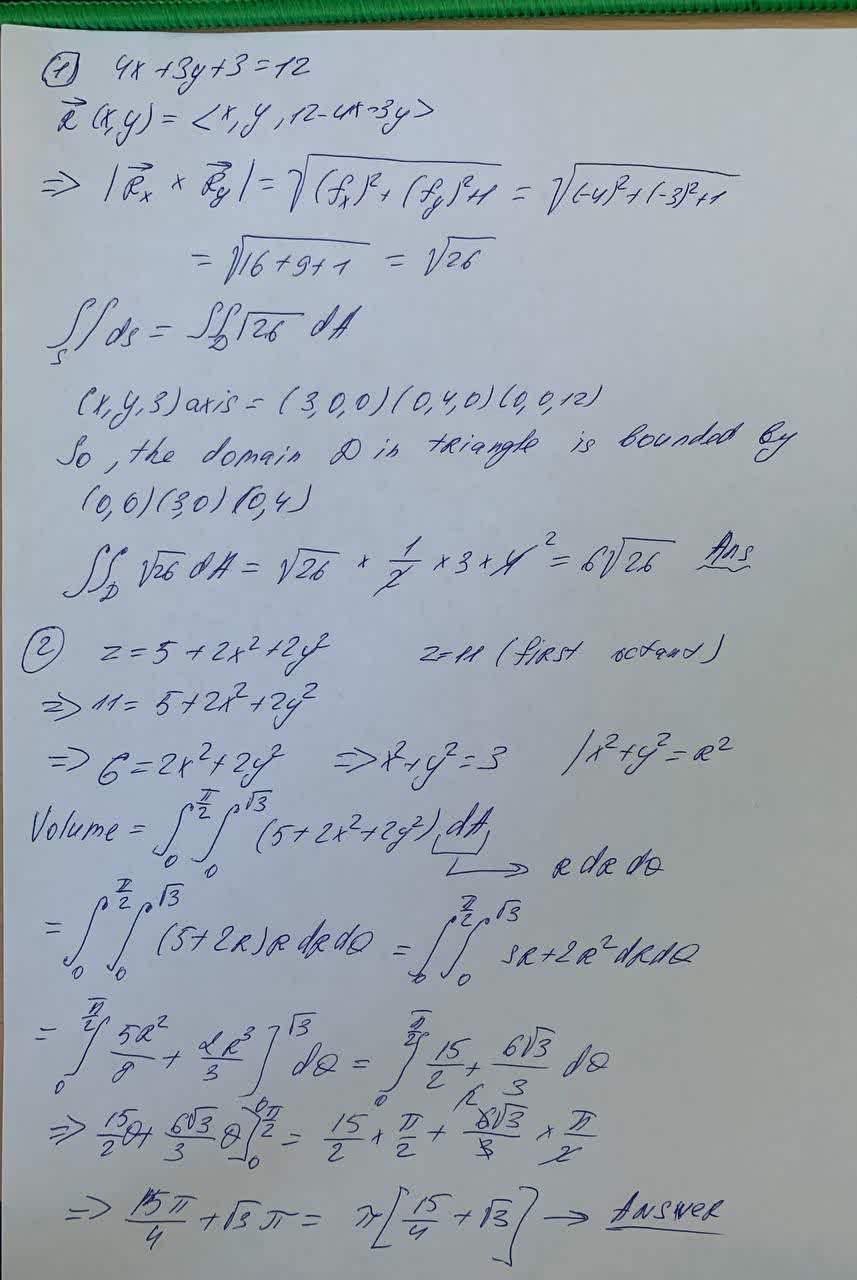# 1) Find the area of the part of the plane4x + 3y + z = 12that lies in the first octant.2) Use polar coordinates to find the volume of the given solid.Bounded by the paraboloid z = 5 + 2x^2 + 2y^2 and the plane z = 11 in the first octantEmeli Hagan 2021-06-08 Answered

1) Find the area of the part of the plane
$$4x + 3y + z = 12$$
that lies in the first octant.
2) Use polar coordinates to find the volume of the given solid.
Bounded by the paraboloid $$z = 5 + 2x^2 + 2y^2$$ and the plane z = 11 in the first octant

• Questions are typically answered in as fast as 30 minutes

### Plainmath recommends

• Get a detailed answer even on the hardest topics.
• Ask an expert for a step-by-step guidance to learn to do it yourself.aprovard###### Have a similar question?content_user

1) $$4x+3y+3=12$$

$$\vec{R}(x,y)=<x,y,12-4x-3y>$$

$$\Rightarrow|\vec{R_x}\times\vec{R_y}|=\sqrt{(f_x)^2+(f_y)^2+1}=\sqrt{(-4)^2+(-3)^2+1}$$

$$=\sqrt{16+9+1}=\sqrt{26}$$

$$\int_S\int ds=\int\int_D\sqrt{26}dA$$

$$(x,y,3)$$ axis $$=(3,0,0)(0,4,0)(0,0,12)$$

So, the domain D in triangle is bounded by $$(0,0)(3,0)(0,4)$$

$$\int\int_D\sqrt{26}dA=\sqrt{26}\times\frac{1}{2}\times3\times4^2=6\sqrt{26}$$

$$z=5+2x^2+2y^2$$

$$\Rightarrow11=5+2x^2+2y^2$$

$$\Rightarrow6=2x^2+2y^2\Rightarrow x^2+y^2=3\ |x^2+y^2=R^2$$

Volume $$=\int_0^{\frac{\pi}{2}}\int_0^{\sqrt{3}}(5+2x^2+2y^2)dA$$

$$=\int_0^{\frac{\pi}{2}}\int_0^\sqrt{3}(5+2R)RdRdQ=\int_0^{\frac{\pi}{2}}\int_0^{\sqrt{3}}3R+2R^2drdQ$$

$$=\int_0^{\frac{\pi}{2}}[\frac{5R^2}{8}+\frac{2R^3}{3}]_0^{\sqrt{3}}dQ=\int_0^{\frac{\pi}{2}}\frac{15}{2}+\frac{6}{\sqrt{3}}dQ$$

$$\Rightarrow\frac{15\pi}{4}+\sqrt{3}\pi=\pi[\frac{15}{4}+\sqrt{3}]$$ Answer